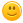# How to make a program, which a bot will quiz us?

asked Nov 24, 2020

Can we make a bot quiz us? How to scan, and reply to us is it wrong/correct?+1 vote
answered Nov 24, 2020 by (560 points)

I don't know how to make a quiz botcommented Nov 24, 2020 by (560 points)
Really Bad. It is really bad to not know
answered Nov 25, 2020 by (58,020 points)
edited Nov 25, 2020 by Peter Minarik

# Start Simple

You can start with something simple as below and upgrade it along the way:

```#include <stdio.h>
#include <string.h>

int main()
{
char capital;
printf("What is the capital of Switzerland? : ");
scanf("%s", capital);
if (strcmp(capital, "Bern") == 0)
{
printf("Correct.\n");
}
else
{
printf("Wrong.\n");
}

return 0;
}```

# Generalise

You can make the whole process generic easily by creating an array of quiz and asking and evaluating each one of these quiz questions.
```#include <stdio.h>
#include <string.h>

#define ArrayLength(array) (sizeof(array) / sizeof(array))

typedef struct
{
const char * question;
const char * answer;
} Quiz;

static void AskQuiz(const Quiz * quiz)
{
printf("%s : ", quiz->question);
{
printf("Correct.\n\n");
}
else
{
printf("Wrong.\n\n");
}
}

int main()
{
Quiz quiz[] =
{
{ "What is the capital of Switzerland?", "Bern" },
{ "What year did World War 2 start?", "1939" },
{ "How high is the highest mountain on Earth (in meters)?", "8848" },
};

int count = ArrayLength(quiz);

for (int i = 0; i < count; i++)
{